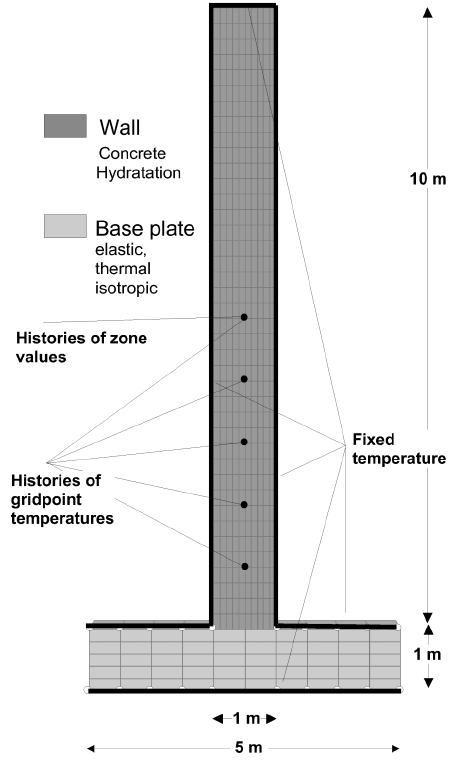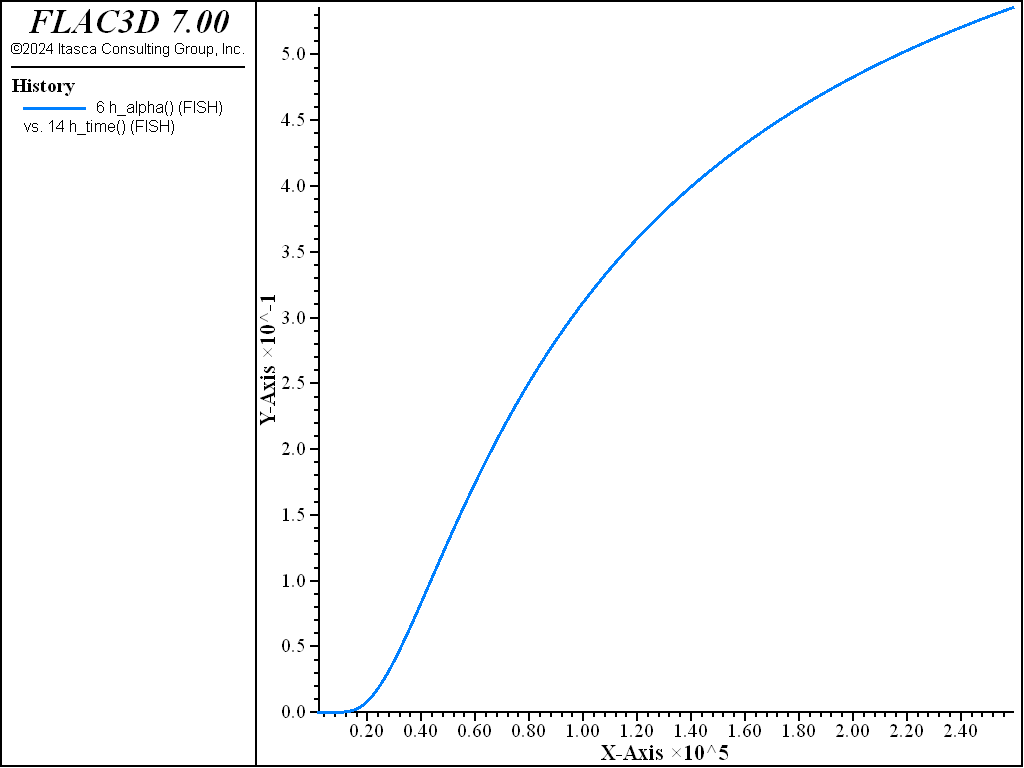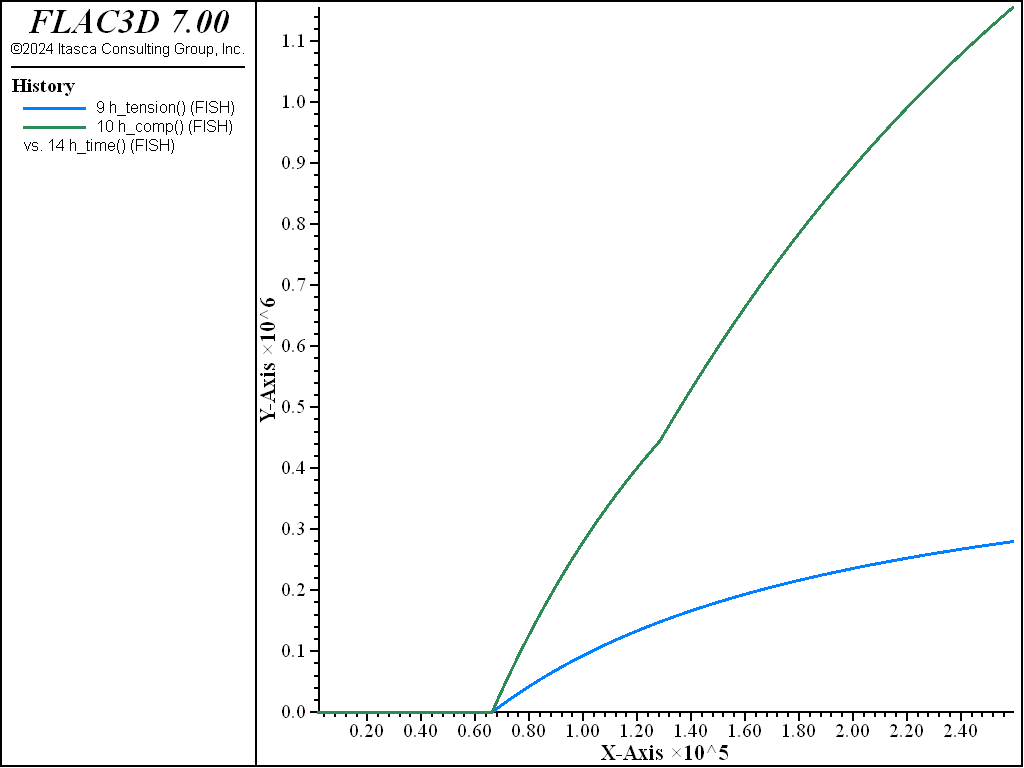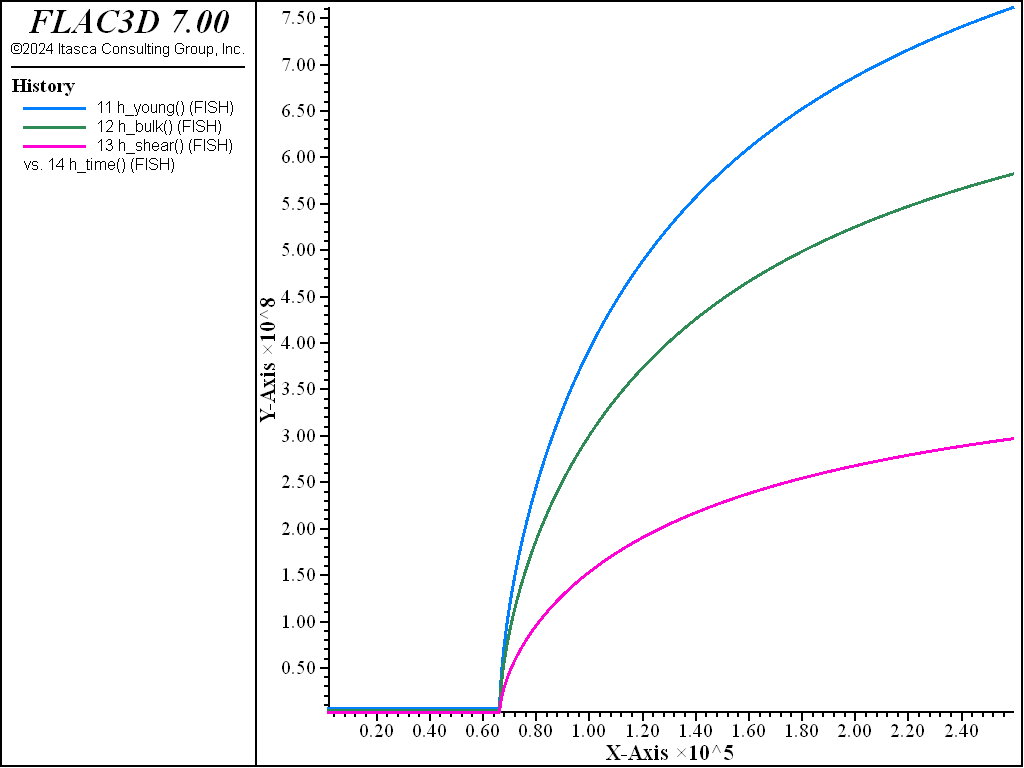# Concrete Wall on Elastic Base Plate

Note

To view this project in FLAC3D, use the menu command Help ► Examples…. Choose “Thermal/ConcreteWall” and select “ConcreteWall.f3dprj” to load. The project’s main data files are shown at the end of this example.

The concrete wall geometry shown in Figure 1 is used for this example. The wall is based upon a model that was developed by Onken and Rostásy (1995) to compare numerical temperature results to temperature measurements. The model consists of a wall of new concrete (10 × 1 × 40 elements) footed on a base plate of old concrete (10 × 1 × 5 elements). The material parameters and boundary conditions are not available from Onken and Rostásy (1995). Therefore, the material parameters from the example of concrete inclusion are used in addition to typical temperature boundary conditions.

The thermal hydration model (hydration) and the mechanical hydration Drucker-Prager model (hydration-drucker-parger) are prescribed for the concrete wall. The base plate is considered elastic and thermally isotropic.

The model is considered to be under summer temperature conditions. The temperatures at the wall boundary are fixed to air temperature; the temperatures at the base plate are fixed to the initial ground temperature:

• initial wall temperature of 293 K
• wall boundary temperature fixed at 293 K
• initial base plate temperature of 285 K
• base plate boundary temperature fixed at 285 K

The numerical results are displayed in Figure 2 to Figure 8: the temperature distribution inside the model after 1 and 3 days (Figure 2 and Figure 3); evolution of gridpoint temperatures (Figure 4); evolution of the hydration grade (Figure 5); evolution of the strength parameters (Figure 6); evolution of the elastic parameters (Figure 7); and evolution of the hydration heat (Figure 8). The results are qualitatively in good agreement with the results given by Onken and Rostásy (1995). (A more detailed comparison is not available due to limited access to material parameters and boundary conditions.)Figure 1: Model of the concrete wall on an elastic base plate.Figure 2: Temperature distribution after one day (concrete age).Figure 3: Temperature distribution after three days (concrete age).Figure 4: Evolution of gridpoint temperatures for the concrete inclusion test as a function of the concrete age.Figure 5: Evolution of the hydration grade as a function of the concrete age.Figure 6: Evolution of the tensile and compressive strengths as a function of the concrete age.Figure 7: Evolution of the elastic parameters as a function of the concrete age.Figure 8: Evolution of the generated hydration heat as a function of the concrete age.

Reference

Onken, P., and F. Rostásy. Wirksame Betonzugfestigkeit im Bauwerk bei früh einsetzendem Temperaturzwang, DAfStb Heft 449. Berlin: Beuth-Verlag (1995).

Data File

;--------------------------------------
; Concrete wall on elastic base plate
;--------------------------------------
model new
model large-strain off
model title ...
"Concrete wall on elastic base plate - summer temperature conditions"
model configure thermal
;
zone create brick point 0=(-0.5,0.0,0.0)  point 1=(0.5,0.0,0.0)   ...
point 2=(-0.5,0.2,0.0)  point 3=(-0.5,0.0,10.0) ...
size = 10,1,100
zone create brick point 0=(-2.5,0.0,-1.0) point 1=(2.5,0.0,-1.0)  ...
point 2=(-2.5,0.2,-1.0) point 3=(-2.5,0.0,0.0)  ...
size = 20,1,5
zone attach by-face range position-z 0
;
zone group 'concrete'    range position-z  0 100
zone group 'base_plate'  range position-z -100 0
;
zone cmodel assign elastic                  range group 'base_plate'
zone thermal cmodel assign isotropic        range group 'base_plate'
zone cmodel assign hydration-drucker-prager range group 'concrete'
zone thermal cmodel assign hydration        range group 'concrete'
;
zone property density=2000 bulk=1e9 shear=0.7e9
zone thermal property conductivity=20. expansion=1e-4  specific-heat=0.2
zone thermal property conductivity= 2. expansion=1e-5  range group 'concrete'
; Modified Drucker-Prager
zone property bulk-reference=0.98e9  shear-reference=0.50e9 ...
range group 'concrete'
zone property constant-c=0.4 constant-a=0.6 ...
range group 'concrete'
zone property hydration-minimum=0.20 tension-reference=2.0e6 ...
range group 'concrete'
zone property hydration-difference-minimum=1e-4 ...
range group 'concrete'
; Thermal hydration
zone thermal property conductivity=2.0 expansion=1e-5 specific-heat=0.2 ...
range group 'concrete'
zone thermal property density-cement=330 constant-jonasson-b=-1.114 ...
constant-jonasson-t1=7.2e4 ...
range group 'concrete'
zone thermal property constant-gas=8.314 temperature-reference=293 ...
range group 'concrete'
zone thermal property constant-energy-1=33.5 constant-energy-dt=1.47 ...
constant-energy-halftime=273 flag-law=0 constant-heat-1=0.2 ...
range group 'concrete'
zone thermal property hydration-binding-maximum=1e5 ...
constant-conductivity-1=2.0 ...
range group 'concrete'
;
zone gridpoint initialize temp 293 range group 'concrete'
zone gridpoint fix temp 293 range position-x -0.5
zone gridpoint fix temp 293 range position-x  0.5
zone gridpoint fix temp 293 range position-z  10.0
zone gridpoint fix temp 285 range group 'base_plate' ; <-- overwrite whatever
zone gridpoint fix velocity   range position-z -1.0
zone gridpoint fix velocity-x range union position-x -2.5 position-x  2.5
;
zone history temperature position (0,0.1,1)
zone history temperature position (0,0.1,2)
zone history temperature position (0,0.1,3)
zone history temperature position (0,0.1,4)
zone history temperature position (0,0.1,5)
[hzp = zone.near(0,0,5)]
fish define h_alpha
global h_q       = zone.therm.prop(hzp,'hydration-rate')
global h_te      = zone.therm.prop(hzp,'age-concrete-effective')
global h_tension = zone.prop(hzp,'tension')
global h_comp    = zone.prop(hzp,'compression')
global h_young   = zone.prop(hzp,'young')
global h_bulk    = zone.prop(hzp,'bulk')
global h_shear   = zone.prop(hzp,'shear')
global h_time    = thermal.time.total
end
fish history h_alpha
fish history h_q
fish history h_te
fish history h_tension
fish history h_comp
fish history h_young
fish history h_bulk
fish history h_shear
fish history h_time
zone geometry-update 10
model solve   time-total [24*3600]
model save 'hyd_exp2_1d'
model solve   time-total [3*24*3600]
model save 'hyd_exp2_3d'